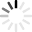Loading VESLUse the slider to linearly combine the (Shapiro and Ritzwoller, 2004) and the (Fox Maule et al, 2005) geothermal heat flux distributions according to the following equation:

G = (1 - α) · GSha + α · GFox

The resulting geothermal heat flux is then used for a thermal steady-state simulation of the Greenland Ice Sheet (upon clicking RUN), with outputs including melt rates and temperature.

The range of values is 0 to 1; 0.5 is used for the initial computation.

The available outputs are melt rate M, melt rate change ΔM, temperature T, and temperature change ΔT at the ice/bed interface.

Because the changes are difficult to visualize, we advise looking at ΔM and ΔT first.

## Info

### Introduction

This simulation of Greenland is the result of work carried out by Dr. Seroussi, Dr. Ivins and Dr. Larour to understand the sensitivity of the thermal regime to geothermal heat flux at the ice/bed interface. The setup relies on the SeaRISE experiments (Nowicki et al. I, 2013, Nowicki et al. II, 2013), and two geothermal heat fluxes distributions from Shapiro and Ritzwoller, 2004 and Fox Maule et al, 2005. Both distributions are used to carry out a steady-state thermal simulation of the temperature and melt rates at the ice/bed interface, and the influence of geothermal heat flux on them.

### Model Settings

• Initial basal friction coefficient inverted from surface velocities (Joughin et al., 2010).
• 3D Steady-State simulation of ice flow, in order to compute internal deformation, needed for strain related heating in the ice volume. Done once, not controllable in this simulation. 3D Stress-Balance using the Shelfy Stream Approximation (MacAyeal et al., 1993).
• 3D Steady State simulation of the thermal regime, using the Aschwanden et al, 2012 enthalpy formulation implemented in ISSM according to Seroussi et al, 2013.
• The slider controls the linear combination of geothermal heat flux distributions according to the following formula: G = (1 - α) · GSha + α · GFox where α ranges from 0 to 1, GSha and GFox are geothermal heat fluxes from Shapiro and Ritzwoller, 2004 and Fox Maule et al, 2005 respectively.

### How to Run the Simulation

To start the simulation, choose a linear combination of both geothermal heat flux distributions (α = 0, Shapiro and Ritzwoller, 2004, α = 1, Fox Maule et al, 2005) using the slider, then click the "RUN" button. Results will be computed by the JPL ISSM server, downloaded, and displayed. Outputs include melt rate change, melt rate, temperature change and temperature at the ice/bed interface.

### Feedback

If you have any questions or feedback, please send us an email.